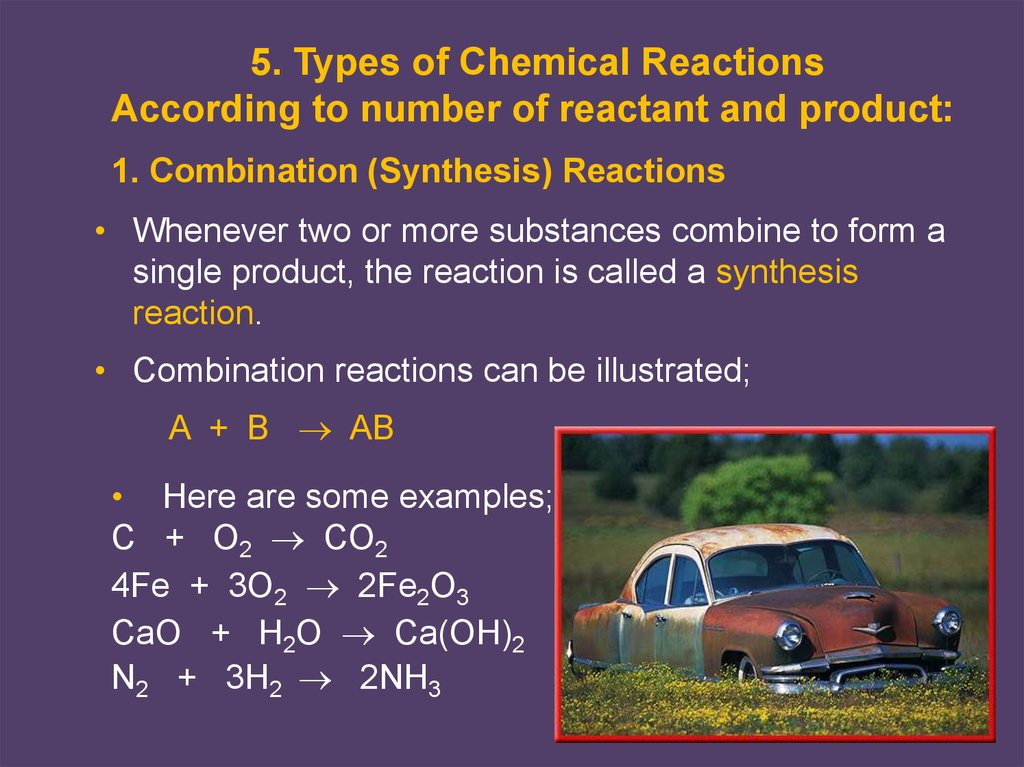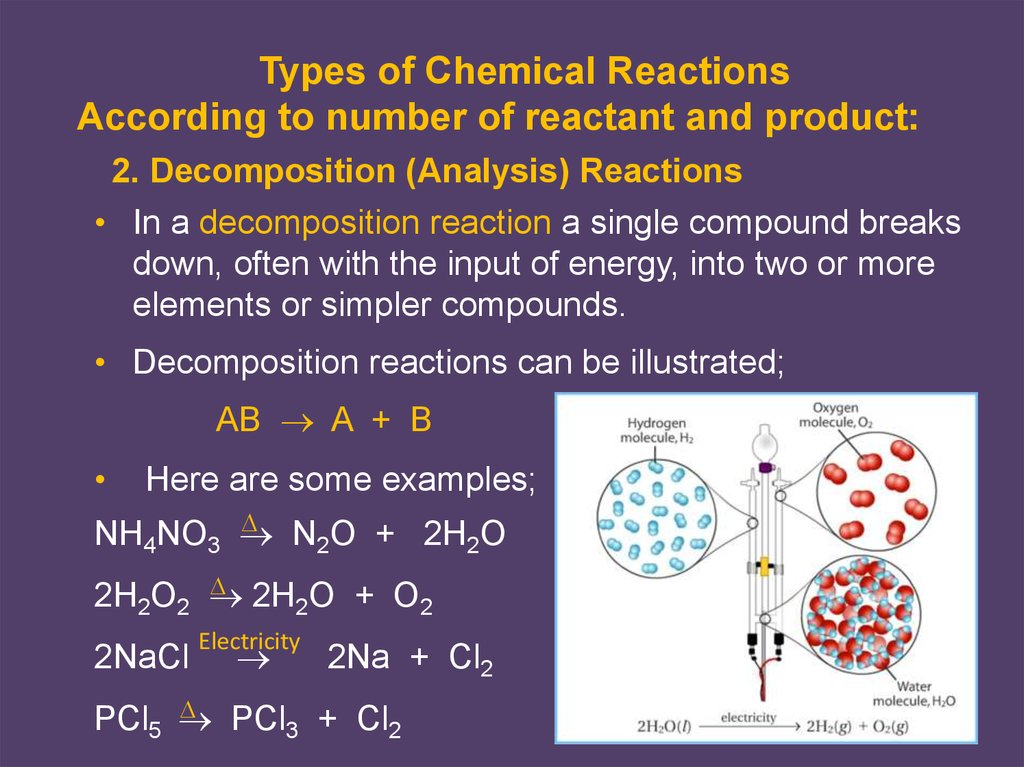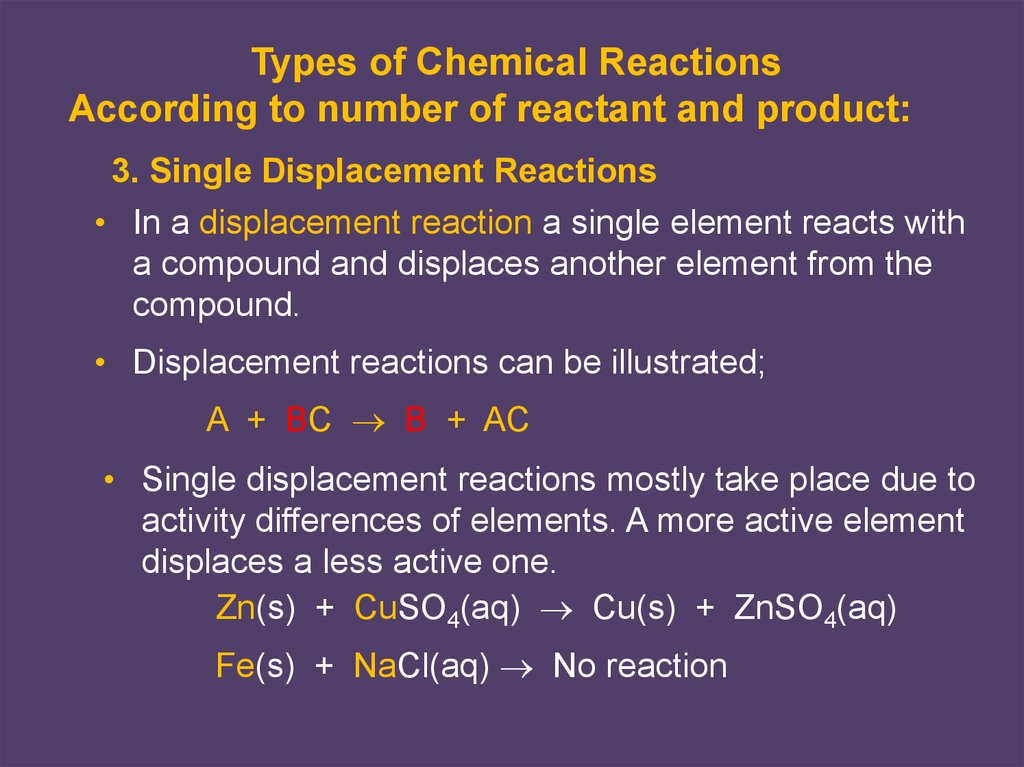# Chemical reactions. (Chapter 5)

## 1. Slayt 1

5. Types of Chemical Reactions
According to number of reactant and product:
1. Combination (Synthesis) Reactions
• Whenever two or more substances combine to form a
single product, the reaction is called a synthesis
reaction.
• Combination reactions can be illustrated;
A + B AB
• Here are some examples;
C + O2 CO2
4Fe + 3O2 2Fe2O3
CaO + H2O Ca(OH)2
N2 + 3H2 2NH3

## 2. Slayt 2

Types of Chemical Reactions
According to number of reactant and product:
2. Decomposition (Analysis) Reactions
• In a decomposition reaction a single compound breaks
down, often with the input of energy, into two or more
elements or simpler compounds.
• Decomposition reactions can be illustrated;
AB A + B
Here are some examples;
NH4NO3
N2O + 2H2O
2H O + O
2H2O2
2
2
2NaCl
Electricity
2Na + Cl2
PCl + Cl
PCl5
3
2

## 3. Slayt 3

Types of Chemical Reactions
According to number of reactant and product:
3. Single Displacement Reactions
• In a displacement reaction a single element reacts with
a compound and displaces another element from the
compound.
• Displacement reactions can be illustrated;
A + BC B + AC
• Single displacement reactions mostly take place due to
activity differences of elements. A more active element
displaces a less active one.
Zn(s) + CuSO4(aq) Cu(s) + ZnSO4(aq)
Fe(s) + NaCl(aq) No reaction

## 4. Slayt 4

Chapter 6
5. Types of Chemical Reactions
3. Single Displacement Reactions, continued
Activity of Some Metals
Li Rb K Ba Sr Ca Na Mg Al Mn Zn Cr Cd Fe
Co Ni Sn Pb H Cu Hg Ag Pt Au
Activity Decreases
Activity of Some Nonmetals
F Cl Br I Activity Decreases
• Some examples;
NaBr(aq) + Cl2(g) 2NaCl(aq) + Br2(g)
Ca(s) + 2H2O Ca(OH)2(aq) + H2(g)
Mg(s) + 2CuCl(aq) MgCl2(aq) + 2Cu(s)

## 5. Slayt 5

Types of Chemical Reactions
According to number of reactant and product:
4. Double Displacement (Metathesis) Reactions
• In a double-displacement reaction two compounds in
aqueous solution appear to exchange ions and form two
new compounds.
• One of the products must be a solid precipitate, a gas,
or a molecular compound, such as water.
• Double displacement reactions can be illustrated;
AB + CD AD + CB
Some examples;
HCl(aq) + NaOH(aq) HOH(l) + NaCl(aq)
Pb(NO3)2(aq) + K2CrO4(aq) PbCrO4(ppt) + 2KNO3(aq)

## 6. Slayt 6

Types of Chemical Reactions
According to heat:
Endothermic and Exothermic Reactions
• A reaction which absorbs energy is endothermic reaction
and which release energy is exothermic reaction.
Some examples;
Ca(s) + 2H2O(l) Ca(OH)2(aq) + H2(g) + Energy
Exothermic Reaction
H2O(l) + Energy H2(g) + O2(g)
Endothermic Reaction

## 7. Types of Chemical Reaction According to states of substance:

1. Homogenous reactions:Reactants and
products are in same state.
N2(g) + H2(g) → NH3(g)
2. Heterogenous reactions: States are different.
Fe(s) + CuCl2(aq) → FeCl2(aq) + Cu(s)
Na(s) + C2H5OH(aq) →C2H5ONa(aq)+H2(g)

## 8. Slayt 8

Types of Chemical Reactions
Combustion Reactions
A combustion reaction is a reaction of a carbon-based
compound with oxygen.
Some examples;
C3H8 + 5O2 3CO2 + 4H2O + heat
CH3CH2OH + 3O2 2CO2 + 3H2O + heat
C6H12O6 + 6O2 6CO2 + 6H2O + heat